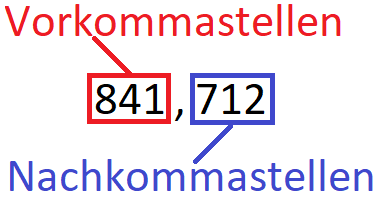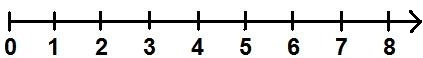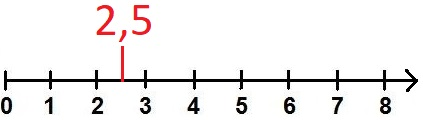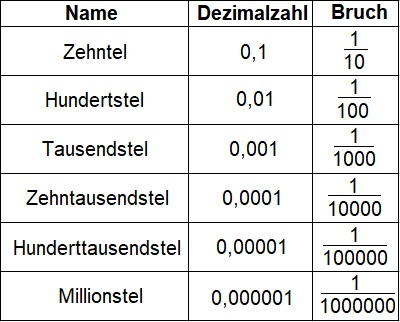# How to Compare Decimal Places

## Decimal numbers (point numbers) definition

What are decimal numbers (point numbers)? How are decimal numbers structured? Let's look at this:

• A Explanation and definition of what decimal numbers and point numbers are.
• Lots Examples to decimals.
• Tasks / exercises so that you can practice this topic yourself.
• A Video to this topic.
• A Question and answer area to this area.

Note: We'll look at decimal numbers in a moment. These are colloquially called floating point numbers. In the following article we will use both names (but there is no difference).

### Decimal numbers: definition and explanation

First, let's take a look at what a decimal is all about. Definition of decimal numbers:

Note:
• A decimal is a number that has a comma.
• Decimal numbers are used to represent non-whole numbers, i.e. parts of something whole.
• A distinction is made between the places before and after the comma.
• These are therefore also called places before and after the decimal point.
• The places to the right of the comma are also called decimal places.

The structure of a decimal number looks like this:Why do you need such point numbers? Perhaps one or the other still remembers the natural numbers: 1, 2, 3, 4, 5, 6 etc. These were already shown on a number line in elementary school:But what happens if you don't want to represent 1 or 2, but something in between? If you have more than 2 cakes but less than 3 cakes. So 2 whole cakes, but only half of a third cake. In this case you can use decimal numbers for representation in mathematics. In this case it would be 2.5.Display:

### Floating number designation

In this section we look at designations for decimal places. More information on basic arithmetic operations and rounding decimal numbers follows.

Examples of decimal numbers:

The next table shows the names of the places after the comma. Thus, the tenth place is directly after the decimal point. The hundredth place is the second place after the decimal point and so on. The last column then shows the representation as a fraction (see fractions):Examples comparing decimal numbers:

Order the following numbers by size, the smallest number first.

• 3,51
• 3,25
• 3,2
• 3,48
• 3,99
• 3,95

Solution:

All numbers have 3 whole. There are no differences in size before the decimal point. For this reason, let's look at the digits after the comma. On the tenth place - i.e. the place directly after the decimal point - we have the smallest number with the 2 (2 numbers have a 2 after the decimal point). Then there is a 4, a 5 and the 9 (twice). So first we have:

This is not quite true yet. Because we still have a hundredth digit. Let's look at these now. We first compare 3.25 and 3.2 with each other. We can write 3.2 as 3.20 and 0 is less than 5. Therefore 3.2 is less than 3.25. Even with 3.99 and 3.95 the order is not correct, since 5 is less than 9.

We obtain:

• 3,2
• 3,25
• 3,48
• 3,51
• 3,95
• 3,99

You can also write this like this:

• 3,2 < 3,25 < 3,48 < 3,51 < 3,95 < 3,99

Show:

### What are decimals?

The next video answers these questions:

• What are decimals / point numbers?
• How do you add decimals?
• How do you subtract point numbers?
• How do you multiply decimal numbers?
• How do you divide point numbers?

Next video »